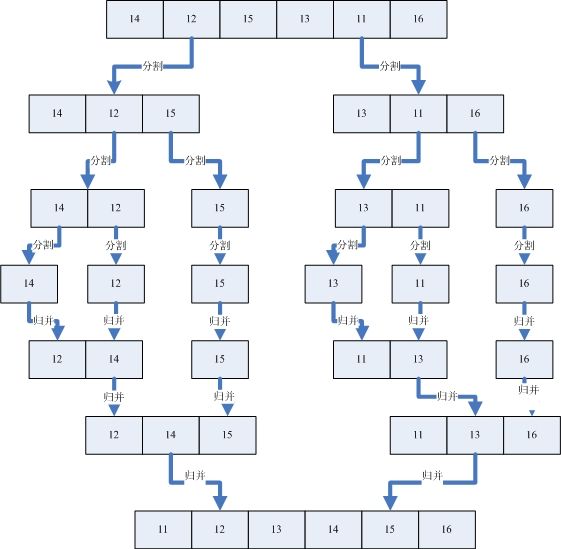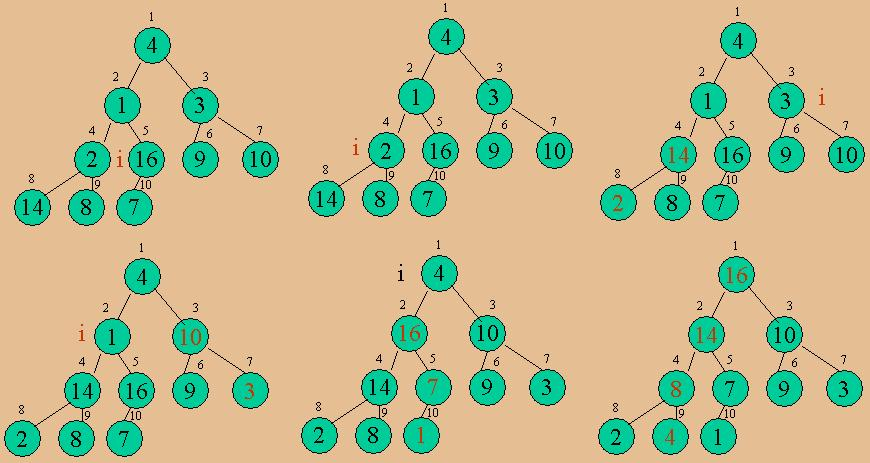# Feeling

三人行，必有我师焉

::  :: 新随笔 :: 联系 ::  :: 管理 ::85 随笔 :: 0 文章 :: 392 评论 :: 0 Trackbacks

1 归并排序（MergeSort）（1）首先设定一个新的数列C。
（2）A和B比较，A = 1，B = 2，A < B，那么C = 1
（3）A和B比较，A = 23，B = 2，A > B，那么C = 2
（4）A和B比较，A = 23，B = 13，A > B，那么C = 13
（5）A和B比较，A = 23，B = 14，A > B，那么C = 14
（6）A和B比较，A = 23，B = 87，A < B，那么C = 23
（7）A和B比较，A = 34，B = 87，A < B，那么C = 34
（8）A和B比较，A = 65，B = 87，A < B，那么C = 65
（9）最后将B复制到C中，那么C = 87。归并完成。

public class MergeSorter
{

private static int[] myArray;

private static int arraySize;

public static void Sort( int[] a )
{
myArray
= a;
arraySize
= myArray.Length;
MergeSort();
}

/// <summary>

/// 利用归并的方法排序数组，首先将序列分割

/// 然后将数列归并，这个算法需要双倍的存储空间

/// 时间是O(nlgn)

/// </summary>
private static void MergeSort()
{

int[] temp = new int[arraySize];
MSort( temp,
0, arraySize - 1);
}

private static void MSort(int[] temp, int left, int right)
{

int mid;

if (right > left)
{
mid
= (right + left) / 2;
MSort( temp, left, mid);
//分割左边的序列
MSort(temp, mid+1, right);//分割右边的序列
Merge(temp, left, mid+1, right);//归并序列
}
}

private static void Merge( int[] temp, int left, int mid, int right)
{

int i, left_end, num_elements, tmp_pos;

left_end
= mid - 1;
tmp_pos
= left;
num_elements
= right - left + 1;

while ((left <= left_end) && (mid <= right))
{

if (myArray[left] <= myArray[mid]) //将左端序列归并到temp数组中
{
temp[tmp_pos]
= myArray[left];
tmp_pos
= tmp_pos + 1;
left
= left +1;
}

else//将右端序列归并到temp数组中
{
temp[tmp_pos]
= myArray[mid];
tmp_pos
= tmp_pos + 1;
mid
= mid + 1;
}
}

while (left <= left_end) //拷贝左边剩余的数据到temp数组中
{
temp[tmp_pos]
= myArray[left];
left
= left + 1;
tmp_pos
= tmp_pos + 1;
}

while (mid <= right) //拷贝右边剩余的数据到temp数组中
{
temp[tmp_pos]
= myArray[mid];
mid
= mid + 1;
tmp_pos
= tmp_pos + 1;
}

for (i=0; i < num_elements; i++//将所有元素拷贝到原始数组中
{
myArray[right]
= temp[right];
right
= right - 1;
}
}
}

2 堆排序（HeapSort）

(1)将原始未排序的数据建成一个堆。
(2)建成堆以后，最大值在堆顶，也就是第0个元素，这时候将第零个元素和最后一个元素交换。
(3)这时候将从0到倒数第二个元素的所有数据当成一个新的序列，建一个新的堆，再次交换第一个和最后一个元素，依次类推，就可以将所有元素排序完毕。public class HeapSorter
{

private static int[] myArray;

private static int arraySize;

public static void Sort( int[] a )
{
myArray
= a;
arraySize
= myArray.Length;
HeapSort();
}

private static void HeapSort()
{
BuildHeap();
//将原始序列建成一个堆

while ( arraySize > 1 )
{
arraySize
--;
Exchange (
0, arraySize );//将最大值放在数组的最后
DownHeap ( 0 );  //将序列从0到n-1看成一个新的序列，重新建立堆
}
}

private static void BuildHeap()
{

for (int v=arraySize/2-1; v>=0; v--)
DownHeap ( v );
}

//利用向下遍历子节点建立堆
private static void DownHeap( int v )
{

int w = 2 * v + 1;                     // 节点w是节点v的第一个子节点

while (w < arraySize)
{

if ( w+1 < arraySize )        // 如果节点v下面有第二个字节点
if ( myArray[w+1> myArray[w] )
w
++;                        // 将子节点w设置成节点v下面值最大的子节点

// 节点v已经大于子节点w，有了堆的性质，那么返回
if ( myArray[v] >= myArray[w] )

return;

Exchange( v, w );
// 如果不是，就交换节点v和节点w的值
v = w;
w
= 2 * v + 1;            // 继续向下找子节点
}
}

//交换数据
private static void Exchange( int i, int j )
{

int t = myArray[i];
myArray[i]
= myArray[j];
myArray[j]
= t;
}
}

3 几种O(nlogn)算法的初步比较

 500随机整数 5000随机整数 20000随机整数 合并排序 0.3125 1.5625 7.03125 Shell排序 0.3125 1.25 6.875 堆排序 0.46875 2.1875 6.71875 快速排序 0.15625 0.625 2.8125

/******************************************************************************************
*【Author】：flyingbread
*【Date】：2007年2月2日
*【Notice】：
*1、本文为原创技术文章，首发博客园个人站点(http://flyingbread.cnblogs.com/)，转载和引用请注明作者及出处。
*2、本文必须全文转载和引用，任何组织和个人未授权不能修改任何内容，并且未授权不可用于商业。
*3、本声明为文章一部分，转载和引用必须包括在原文中。
******************************************************************************************/
posted on 2012-11-10 23:18 三人行，必有我师焉 阅读(439) 评论(2)  编辑  收藏### 评论

# re: 排序1+4：归并排序（MergeSort）和堆排序（HeapSort）（转） 2012-11-11 01:52 三人行，必有我师焉

# re: 排序1+4：归并排序（MergeSort）和堆排序（HeapSort）（转） 2012-11-12 01:16 三人行，必有我师焉

1.以数组最右端的元素作为分割点
2.做一个标记符，标记已经放了几个元素到左边了
3.开始遍历数组每个元素，碰到小于分割点的元素，就和第（标记符+1）个元素交换，然后标记符增加1。
4.将分割点和第（标记符+1）个元素交换，这是第（标记符+1）个元素左边的元素都小于分割点，右边的元素都大于或等于分割点元素。
5.递归排序分割点左边的部分和右边的部分，直到子数组的左边部分索引和右边部分索引相等，也就是长度为1为止。  回复  更多评论

 只有注册用户登录后才能发表评论。 网站导航:
GitHub |  开源中国社区 |  maven仓库 |  文件格式转换